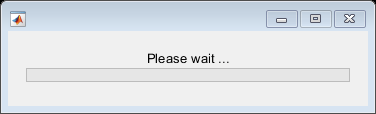# Update Wait Bar While Functions Run in the Background

This example shows how to use `afterEach` to update a wait bar with the progress of functions running in the background.

Create a wait bar, `w`.

`w = waitbar(0,'Please wait ...');`Set the number of iterations for your `for`-loop, `N`. Store the current number of completed iterations, `0`, and the total number of iterations, `N`, in the `UserData` property of the wait bar.

```N =20; w.UserData = [0 N];```

Run a `for`-loop with `N` iterations. In each iteration, use `parfeval` and `backgroundPool` to run `pause` in the background for a random number of seconds. Store each `Future` object in an array.

```for i = 1:N delay = rand; f(i) = parfeval(backgroundPool,@pause,0,delay); end```

Use the helper function `updateWaitbar` to update the waitbar after each `Future` finishes.

`afterEach(f,@(~)updateWaitbar(w),0);`

Use `delete` to close the wait bar after all the `Future` objects finish.

`afterAll(f,@(~)delete(w),0);`

### Define Helper Function

Define the helper function u`pdateWaitbar`. The function increments the first element of the `UserData` property, then uses the vector to calculate the progress.

```function updateWaitbar(w) % Update a waitbar using the UserData property. % Check if the waitbar is a reference to a deleted object if isvalid(w) % Increment the number of completed iterations w.UserData(1) = w.UserData(1) + 1; % Calculate the progress progress = w.UserData(1) / w.UserData(2); % Update the waitbar waitbar(progress,w); end end```